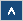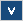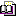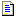Verilog Online HelpTable of ContentsBit-selectBlock StatementsBuilt-in PrimitivesCase StatementContinuous AssignmentsConversion FunctionsCommentsCompiler DirectivesConcatenationsConditional OperatorDelaysDisable StatementDisplay TasksEdge Sensitive PathExpression Bit LengthFile I/O FunctionsFunctionsIdentifiersIf StatementInteger ConstantsIntra-assignment Timing ControlsKeywordsLoop StatementsMemoriesmin:typ:max DelaysModule DeclarationModule InstantiationModule Path DeclarationModule Path PolarityNet Data TypesOperatorsParametersPart-selectPLA Modeling TasksProbabilistic Distribution FunctionsProcedural AssignmentsProcedural Continuous AssignmentsProcedural Timing ControlRange SpecificationReal ConstantsRegister Data TypesSimulation Control TasksSimulation Time FunctionsSpecify BlockState Dependent PathStochastic Analysis TasksStrengthsStringsStructured ProceduresTasksTimescale System TasksTiming Check TasksUDP DeclarationUDP InstantiationUDP State TableValue Change Dump (VCD) FileVectors

# Probabilistic Distribution Functions

## Formal Definition

Probabilistic Distribution Functions provide the functionality of random number generators.

## Simplified Syntax

\$dist_uniform (seed, start, end) ;

\$dist_normal (seed, mean, standard_deviation) ;

\$dist_exponential (seed, mean) ;

\$dist_poisson (seed, mean) ;

\$dist_chi_square (seed, degree_of_freedom) ;

\$dist_t (seed, degree_of_freedom) ;

\$dist_erlang (seed, k_stage, mean) ;

## Description

Probabilistic distribution functions present a way to test your design using a randomly generated data. Testbenches often do not reveal irregularities in working models because designers tend to write testbenches in a schematic fashion. Usually, test vectors applied to the inputs of a module do not cover all possible states. When used, these functions may find a specific input combination for which the model does not work correctly. To enable repetitive design debugging, the probabilistic distribution functions must return recurrent data. This means that each time they are called they should return the same values in the last call order.

Probabilistic distribution functions use several arguments for generating data. A first function argument is a seed. Each time a function is called, the seed argument determines an order of values that are returned from the function. This argument must be a register type value of an inout direction. Users can initialize this value and let the system override it. This will make these functions repetitive.

The \$dist_uniform function returns an integer value that is a random generated number in a range depending on the start and end arguments. Returned values are uniformly distributed. Both start and end arguments can be any integer, positive or negative, however the start value should be smaller than the end value.

The \$dist_normal function returns a number that is an average approach to the mean argument. The mean argument is also used in \$dist_expotential, \$dist_poisson and \$dist_erlang. The standard_deviation argument is used to determine the shape of density. In \$dist_chi_square and \$dist_t the shape of density depends on the degree_of_freedom.

## Examples

Example 1

reg [15:0] a ;
initial begin
a = \$dist_exponential(60, 24);
end

Example 2

reg [15:0] a ;
initial begin
a = \$dist_erlang(60, 24, 7) ;
end

## Important Notes

• To enable repetitive design debugging, the probabilistic distribution functions return recurrent data.